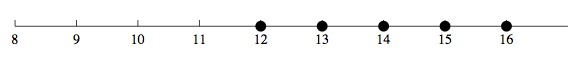# Sports Equipment Set

Alignments to Content Standards: 7.EE.B.4.b

Jonathan wants to save up enough money so that he can buy a new sports equipment set that includes a football, baseball, soccer ball, and basketball. This complete boxed set costs \$50. Jonathan has \$15 he saved from his birthday. In order to make more money, he plans to wash neighbors’ windows. He plans to charge \$3 for each window he washes, and any extra money he makes beyond \$50 he can use to buy the additional accessories that go with the sports box set.

Write and solve an inequality that represents the number of windows Jonathan can wash in order to save at least the minimum amount he needs to buy the boxed set. Graph the solutions on the number line. What is a realistic number of windows for Jonathan to wash? How would that be reflected in the graph?

## IM Commentary

The purpose of this task is to present students with a context that can naturally be represented with an inequality and to explore the relationship between the context and the mathematical representation of that context; thus, this is an intended as an instructional task. For example, some reasoning is needed to see that our solution set will be whole numbers only and will at some point be cut off, as realistically Jonathan does not have, for example, a million windows in his neighborhood.

When the numbers are small enough, a student can easily use repeated addition to come up with the answer. The value of representing it with an inequality is clearer when the numbers are bigger. Simply changing the amount he needs to save to \$100 or so will make this point. ## Solution We wish to find how many windows Jonathan must wash, so we let$w$be the number of windows. As he expects to get \$3 per window, we multiply these two quantities.

$$3w$$

This represents how much money Jonathan will make just from his window washing. Since he already has \$15 saved, we now add 15 to this amount. $$3w + 15$$ Because we know that Jonathan needs a minimum of \$50, but could have more, we set this greater than or equal to 50.

$$3w + 15 \geq 50$$

We can solve this expression by first subtracting 15 from both sides, and then dividing both sides by 3 to isolate $w$.

\begin{align} 3w + 15 &\geq 50 \\ 3w &\geq 35 \\ w &\geq \frac{35}{3} =11\frac23 \end{align}

Since we cannot (or should not) wash just $\frac23$ of a window, it makes sense that we round this number up to 12. Thus, Jonathan must wash at least 12 windows in order to purchase the sports set. Note that this is just the minimum number he must wash, and washing more would be in his benefit, as he can purchase more sports accessories. Using this information, and the fact that only whole numbers make sense in this context, our solution set can be graphed as follows.There are other possible graphs, as the right hand endpoint can be determined based on a discussion based on what would be "realistic" based on, for example, how many neighbors Jonathan has, how many windows are in each house, and how much time he has to wash windows. The point of the question is to have students realize that a context limits the solution set even when other numbers satisfies the accompanying inequality.